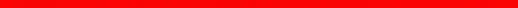Physics Department | Astronomy at UM |  Search

Review Checklist - Sample Questions

Energy, Heat and Temperature

• What is energy?
• What is kinetic energy?
• What is gravitational potential energy?
• In what sense is mass energy?
• What forms of energy do we consider to be internal energy?
• What is thermal energy?

• Does a pen at rest on a desk have kinetic energy? Potential energy? Mass energy?
• Does a baseball in flight have kinetic energy? Potential energy? Mass energy?
• If an object is placed somewhere at rest, will its energy depend on where it is placed?
• When a ball drops from a height and speeds up, what kinds of energy are changing?
• When a fast moving ball crashes through a window, what kinds of energy are changing?
• [When wood burns into ashes and smoke, what kinds of energy change in the process?]
• [How does the Sun produce all the energy we receive on Earth?]

• If thermal energy is motion of particles, why is it different from kinetic energy?
• What happens inside all types of matter when temperature rises?
• Why does matter solidify when cooled down, and not when heated up?
• What is the difference between temperature and heat?
• What is the lowest possible temperature that can be reached?
• Can an object be heated without it changing temperature?page by luca bombelli <bombelli at olemiss.edu>, modified 29 sep 2012